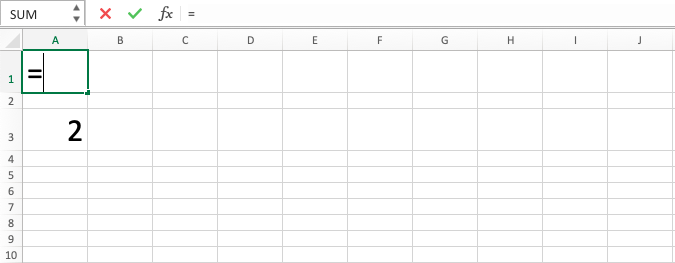Excel INDIRECT Formula - Compute Expert

# Excel INDIRECT Formula

In this tutorial, you will learn the INDIRECT function in excel. Excel INDIRECT formula is useful to get coordinate cell reference from a text data that can be used in your formula writing.

## Why do We Need to Learn About the INDIRECT Function in Excel?

In doing data processing on a spreadsheet, often we need to write a formula so we can do calculation and analysis processes much faster to the data. In the writing, we sometimes need cell coordinates as references in it. The coordinates sometimes cannot be inputted directly, though, and are needed to be written in the form of text first to do the data analysis as we need.

An example is when we do data analysis to analyze and decide promotion activities that are needed for a product in a region. To do this, sometimes we need data history on the product’s performance in the region like how many sales it can get in a period or other data related to the promotion activities that have been done before for the product. But, because of data complexity in the spreadsheet, maybe sometimes it is harder to write the cell coordinate as needed in the formula and it is better if we write it in the form of text first with the help of lookup formulas like MATCH to get the column and row position of the cell coordinate according to the need in the formula.

This, of course, cannot be done by you manually because the spreadsheet does not have that kind of capability. To help you in doing the change from text writing of a cell coordinate to a reference form in a formula, then you can use excel INDIRECT formula.

INDIRECT function in excel can help you to turn a coordinate text data into a cell reference as you need in data analysis. It is very useful if you need this kind of conversion process which is often met in the advanced data analysis in a spreadsheet.

## What is Excel INDIRECT Formula?

Excel INDIRECT formula is a formula with the usage objective to get a reference form from the text data form of a cell coordinate to be used in a formula. INDIRECT function in excel is often used if you still don’t know for sure about the exact cell coordinate to be used and need to do processing first to know it.

In general, the explanation about the INDIRECT function in excel is as follows:

=INDIRECT(ref_text, a1)

Notes:
• ref_text = cell coordinate in text data form to be changed into a reference
• a1 = optional. TRUE or FALSE input to tell the form of text that you want to convert, A1 or R1C1

## How Can You Use the INDIRECT Function in Excel?

The following will explain how the writing of excel INDIRECT formula is done. It needs one required input which is the text form of the cell coordinate and one optional input which tells the text form that you want to convert for the result.

## Using Excel INDIRECT Formula

1. Type equal sign ( = ) in the cell where you want to put the result in2. Type INDIRECT (can be with large and small letters) and open bracket sign after =3. Type cell coordinate in text form or cell coordinate where that text is after open bracket sign4. Optional: Type a comma sign ( , ) and input TRUE or FALSE as the text form inputted earlier that you want to convert. TRUE means A1 and FALSE means R1C1. If you don’t give input here, then it will be considered as TRUE5. Type close bracket sign6. Press Enter
7. The process is done!## Exercise

After you have understood how to use the excel INDIRECT formula from the tutorial above, you can practice your understanding by doing the exercise below!

Download the exercise file and do all the questions. Download the answer key file to check if you have done the exercise or use it if you are confused about how to do the exercise!

### Instructions

1. Refer to B3 cell content in C3!
2. Sum B4 reference with B3!
3. Multiply B5 reference with B4!

INDIRECT function in excel is often combined with ADDRESS because ADDRESS can produce a cell coordinate in the form of text from the row and column number inputted in it. Learn ADDRESS also if you want to use INDIRECT function in excel optimally!

Get updated excel info from Compute Expert by registering your email. It's free!